PHYSICS

Mass

FUNDAMENTAL OF PHYSICS

FA. FUNDAMENTAL OF PHYSICS

1. Dimensional Analysis 2. Plank Constant and Photon 3. Mass

INTRODUCTION

The fundamental of Physics is the basic principle to understand about the Physics. For easier and better understanding of physics, it is dissected into the most fundamental dimensions. The most fundamental dimensions are the basic ingredients of Physics. By knowing and understanding about the ingredients, we can see clearly the way of Physics in governing the Universe. We begin with the dimension analysis by defining the most fundamental dimensions or the ingredients.

OBJECTIVES

1)      To discuss the most fundamental dimensions of Physics.

2)      To discuss and dissect the multi-dimensional constants.

3)      To find and develop the fundamental of mass.

FA.1.0        DIMENSIONAL ANALYSIS

Dimensional analysis is one way to understand the characteristic of something fundamentally. There are 4 ultimate and most fundamental dimensions appear in this universe. These fundamental characteristics are carried by the electromagnetic wave. The ingredients are,

1)      Charge/Electric, represented by symbol [Q]

2)      Magnetic, represented by symbol, [B]

3)      Length, represented by symbol, [L] and

4)      Time, represented by symbol, [T]

As shown by Maxwell and his magnetron, wave is induced and generated through oscillating the magnetic and electric (M&E) field. Wave travels at the speed of light. Wave carries the characteristic of the M&E field, length and time.

## FA.1.1        Multi-Dimensional Constants

This chapter discusses about multi-dimensional constants and their meanings which represented by their dimensions. In our case, only 4 constants are selected due to their representing characteristic. They are listed as follows,

1)      Permittivity Constant, εo = 8.85 x 10-12 F/m

2)      Permeability Constant, μo = 1.26 x 10-6 H/m (T.m/A)

3)      Plank Constant, h = 6.63 x 10-34 J.s

4)      Speed of Light, c = 3.0 x 108 m/s

Each of the constant above carries different meaning. The permittivity constant relates to the electric, the permeability constant relates to the magnetic, the plank constant relates to the photon energy and the speed of light relates to the time-space. These 4 constants are selected because they carry the ingredients of the universe.

The above constants are multi-dimensional and will be dissected into the fundamental dimensions. Along the process of dissecting the multi-dimensional constants, we need to resolve for another intermediate dimension, which is mass, [M]. Mass is not a fundamental dimension.

#### FA.1.2        Dissection of Permittivity Constant

The permittivity constant has the dimension of F/m, which can be dissected as follows,… eq. FA.1.1

where   [N] = Newton

The dimension for [N] is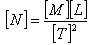… eq. FA.1.2

Substitute eq. FA.1.2 into eq. FA.1.1, we obtain,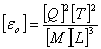… eq. FA.1.3

To the fundamental dimension, the permittivity constant is a function of electric, mass, length, and time.

#### FA.1.3        Dissection of Permeability Constant

The permeability constant has the dimension of H/m, which can be dissected as follows: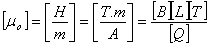… eq. FA.1.4

where   A = ampere, has the dimension of charge over time, [Q]/[T]

T = Tesla, has the dimension of magnetic, [B]

To the fundamental dimension, the permeability constant is a function of magnetic, electric, length, and time.

#### FA.1.4        Dissection of Speed of Light

The dimension of speed of light is very straight forward and simple as follows,

[c] = [L] / [T]                                                                                       … eq. FA.1.5

The speed of light is a function of length, and time.

#### FA.1.5        Dissection of Mass

Before we further proceed with the dimensional dissection on the Plank constant, we need to resolve the intermediate dimension, mass. Mass is not a fundamental dimension.

Multiplying eq. FA.1.3 and eq. FA.1.4, we obtain,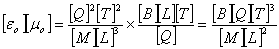… eq. FA.1.6

We also know that the relation between the speed of light, the permittivity and permeability constants is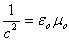… eq. FA.1.7

Inserting eq. FA.1.6 into eq. FA.1.7, we obtain,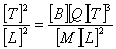… eq. FA.1.8

Rearranging eq. FA.1.8 and solving for mass, we obtain,

[M] = [B][Q][T]                                                                                   … eq. FA.1.9

From eq. FA.1.9, we can summarize that mass is a function of magnetic, electric and time. This functionality is very important for us to understand the mass. These are the ingredients that made up the mass. From this fundamental, a new identity of mass is introduced and discussed in the topic ‘Mass’.

#### FA.1.6        Dissection of Plank Constant

The plank constant relates to the light energy. It has the dimension of J.s, which can be dissected as follows,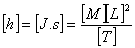… eq. FA.1.10

FA.1.7        Summary of The 4 Ultimate Constants

From the above dissections, we can summarize the 4 multi-dimensional constants to the 4 fundamental dimensions by inserting eq. FA.1.9 into eq. FA.1.3 and eq. FA.1.10 as follows,

1)      Permittivity Constant, [εo] = [Q][T] / [B][L]3

2)      Permeability Constant, [μo] = [B][L][T] / [Q],

3)      Plank Constant, [h] = [B][Q][L]2, and

4)      Speed of Light, [c] = [L] / [T]

We can express the above 4 constants as follows,

1)      Permittivity constant is a function of magnetic, electric, length, and time.

2)      Permeability constant is a function of magnetic, electric, length, and time.

3)      Plank constant is a function of magnetic, electric and length.

4)      Speed of Light is a function of length and time.

FA.2.0        PLANK CONSTANT AND PHOTON

The Plank constant contains very useful information about the photon. This functionality is very important to understand and explain everything about the photon. These are the ingredients that made up the photon. From this fundamental, a new identity of photon is introduced and discussed in the topic “Wave and Photon”. The mechanism on the refraction, reflection, diffraction and interference of photons are presented.

As proven by the Faraday’s law and Maxwell’s law of induction, photon is the energy oscillating in the M&E field which perpendicular to each other. This phenomenon is the perfect and neutral condition. Therefore, photon does not possess the charge and magnetic dipole properties. However, due to the M&E property of photon, the photon will react upon the external M&E field.

For discussion purposes, we summarize some important features about the photon as follows,

Photon refers to all ranges of the electromagnetic wave. Photon is the kinetic-time-energy at time zero. As proven by the Maxwell’s law and his magnetron, wave is produced by oscillating the M&E field. Also as shown by Einstein, photon carries energy and travels at the light speed. Photon energy is quantized according to the Plank’s constant. Photon possesses the characteristic of the magnetic and electric. Photon is the ‘death’ time-energy; the frequency of photon represents its energy level.

FA.3.0        MASS

From the chapter FA.1.0, eq. FA.1.9 as quoted below; the ingredient of the mass is the magnetic and electric (M&E) field and time. It is formed under the present of rapid changing of the M&E field with the ‘excess’ of time during the big bang of the Universe. Mass is the potential-time-energy. Mass possesses its ‘volumetric’ size due to the M&E field.

[M] = [B][Q][T]                                                                                   … eq. FA.1.9

Mass is the function of the M&E field and time. When time prolongs, the mass undergoes decay process to release the stored time-energy back to the universe, in the form of electromagnetic wave. Mass is the potential-time-energy. Wave is the kinetic-time-energy. The decay process is the energy transformation process where the potential energy is converted to the kinetic energy.

Also as shown by Einstein, E=mc2=hf, where the decaying of mass produces photons. Therefore, we can make a reverse conclusion that the mass is made up of the photon. Since the photon contains the M&E property, the mass shall also contain the M&E property. Both possess the same ingredients.

In short, we can conclude that the mass has nothing except the M&E field. The difference between the photon and the mass is, the mass possesses another property, which is the stored-time.

For discussion purposes, we summarize some important features about the mass as follows,

Mass refers to all kinds of objects and things that formed by particles, atoms or electrons. Mass is a function of magnetic, electric and time. Mass is the potential-time-energy which produced during the formation of the Universe. When time prolongs, the 'excess' time of mass undergoes decay process releasing the stored time-energy back to the Universe, in the form of electromagnetic wave. Mass is the counter-part of photon. Both mass and photons consist of the same ingredients which is the magnetic and electric fields.

FA.3.1        ‘Volumetric’ of Mass

From the eq. FA.1.9, it is clearly stated that mass is a function of M&E field and time. Mass is not a stuffed or solid object. Mass is just a time-energy. Mass possesses its ‘volumetric’ size due to the M&E field.

For better illustration and understanding, we relate the mass and photon according to the reverse decaying process. Photon is straight traveling. Mass is the twisted photon. The M&E characteristics cannot be separated because each causes each to appear. The twisted M&E field produces the ‘volume’ of the mass or particle. The transformation between the photon and mass will be discussed in more detail in the topics of “Mass” and “Wave and Photon”.

FA.4.0        THEORY OF EVERYTHING

FA.4.1        Dimension of Energy

The energy has the unit Joule, which is described dimensionally as follows,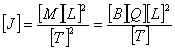… eq. FA.1.11

Energy is the function of the magnetic, electric charge, length and time. Energy is conserved in the Universe. Energy consists of all the 4 fundamental dimensions. These 4 ingredients form the basis of the entire activities in the Universe. The Universe contains nothing but only the charge, magnetic, space and time.

This is the basis for the Theory of Everything. Everything in the Universe can be explained and related to these 4 ingredients.

CONCLUSIONS

From the dimensional analysis, we are able to understand the properties and the characters of the substances. The dissection of the four ultimate multi-dimensional constants discovers the dimension of mass and identifies the property of the photon and mass.

The mass is not the fundamental dimension; it is the function and made up of the magnetic, electric and time. Mass and photon are interchangeable; therefore, both are having the same ingredients, which are the magnetic and electric property. The mass and photon are having a new identity which will be discussed in separate topics.

The four ingredients are the most fundamental substances, which are the electric charge, magnetic, length and time. These four properties govern all the activities in the Universe. The energy possesses all the four ingredients and the energy is conserved all the time.

The four ingredients form the basis for the Theory of Everything.

This website is originated on 15-Mar-2007,

updated on 4-Jan-2009.